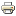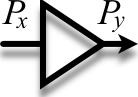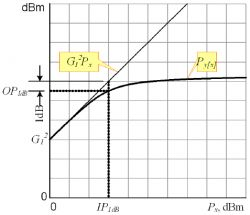One-decibel Compression Point P1dB#### One-decibel Compression Point, P1dBIn the RF design and output stage calculations such parameter as one-decibel compression point (P1dB) is often used. The definition, scope of use, and some notes regarding interconnection with other parameters are described below.

Let x(t) — signal at the input of the system.

x(t)=√2√Px•sin(ω0t),

where Px — mean power of the x(t). Let’s show on a graph in log scale dependence of output power on the input power Px at given frequency ω0, fig. 1.Output power vs. input power, P1dB definition Fig. 1. Output power vs. input power

As would be expected, at low level of input signal the graph coincides with straight line with slope angle tangency equaled to one. This segment of the graph corresponds to the mode of the stage close to linear (incidental nonlinearity). Further as the input power grows, the graph changes its slope and becomes almost flat at the point when the stage enters saturation mode. This segment of the graph corresponds to essential nonlinearity.

It’s clear that power series approximation can be used only if the signal is small enough. Thus there is a need in parameter defining the range of use of approximation and specifying to some extent the maximum output power of the amplifier stage. (There are some tasks when nonlinear distortion is not so important, and the key parameter is being maximum output power, for example, in the case of LO signal driver for passive balanced mixer.)

Such a parameter is one-decibel compression point P1dB. It equals to such output signal power, that difference between asymptotic linear characteristic and actual power equals to 1 dB (fig. 1). As a rule compression point refers to output, so it is denoted as OP1dB or simply P1dB, and IP1dB — when it refers to input. Apparently, from the definition above follows:

OP1dB=IP1dB+(G-1),    [dB],

where G — gain of the amplifier stage (dB).

In practice there is an empiric rule — it’s supposed that the incidental nonlinearity range lays 6 – 10 dB below one-decibel compression point. I.e. the estimation of the nonlinear distortion, originally based on the power series approximation, can be applied only if the signal level is this small. In amplifiers with deep inverse feedback (most of gain blocks) the transition from incidental to essential nonlinearity is quite sharp, so the power in saturation mode (Psat) is only 1 – 1.5 dB above the decibel compression point.

As the compression point is defined for sine signal with specified frequency, so in datasheets P1dB vs. frequency graph could be presented.